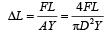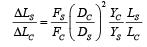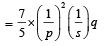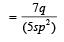Courses

# Test: Past 28 Years Questions: Mechanical Properties of Solids

## 5 Questions MCQ Test Physics Class 11 | Test: Past 28 Years Questions: Mechanical Properties of Solids

Description
This mock test of Test: Past 28 Years Questions: Mechanical Properties of Solids for NEET helps you for every NEET entrance exam. This contains 5 Multiple Choice Questions for NEET Test: Past 28 Years Questions: Mechanical Properties of Solids (mcq) to study with solutions a complete question bank. The solved questions answers in this Test: Past 28 Years Questions: Mechanical Properties of Solids quiz give you a good mix of easy questions and tough questions. NEET students definitely take this Test: Past 28 Years Questions: Mechanical Properties of Solids exercise for a better result in the exam. You can find other Test: Past 28 Years Questions: Mechanical Properties of Solids extra questions, long questions & short questions for NEET on EduRev as well by searching above.
QUESTION: 1

### Two wires A and B are of the same material. Their lengths are in the ratio 1 : 2 and the diameter are in the ratio 2 : 1. If they are pulled by the same force, then increase in length will be in the ratio

Solution:

We know that Young's modulus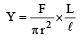Since Y, F are same for both the wires, we have,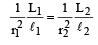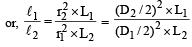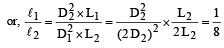So,    ℓ1 : ℓ2 = 1 : 8

QUESTION: 2

### When an elastic material with Young’s modulus Y is subjected to stretching stress S, elastic energy stored per unit volume of the material is

Solution:

Energy stored per unit volume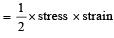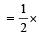stress (stress / Young' s modulus)(stress)2 /( Young ' s modulus)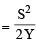QUESTION: 3

### The compressibility of water is 4 × 10–5 per unit atmospheric pressure. The decrease in volume of 100 cm3 of water under a pressure of 100 atmosphere will be

Solution: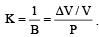Here, P = 100 atm,
K = 4 × 10–5  and V = 100 cm3.
Hence, ΔV = 0.4 cm3

QUESTION: 4

The following four wires are made of the same material. Which of these will have the largest extension when the same tension is applied ?

Solution: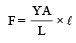So, extension,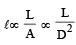[∵ F and Y are constant]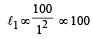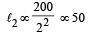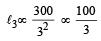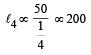The ratio of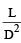is maximum for case (d).
Hence, option (d) is correct.

QUESTION: 5

If the ratio of diameters, lengths and Young’s modulus of steel and copper wires shown in the figure are p, q and s respectively, then the corresponding ratio of increase in their lengths would be [NEET Kar. 2013]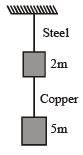Solution:

From formula, Increase in length